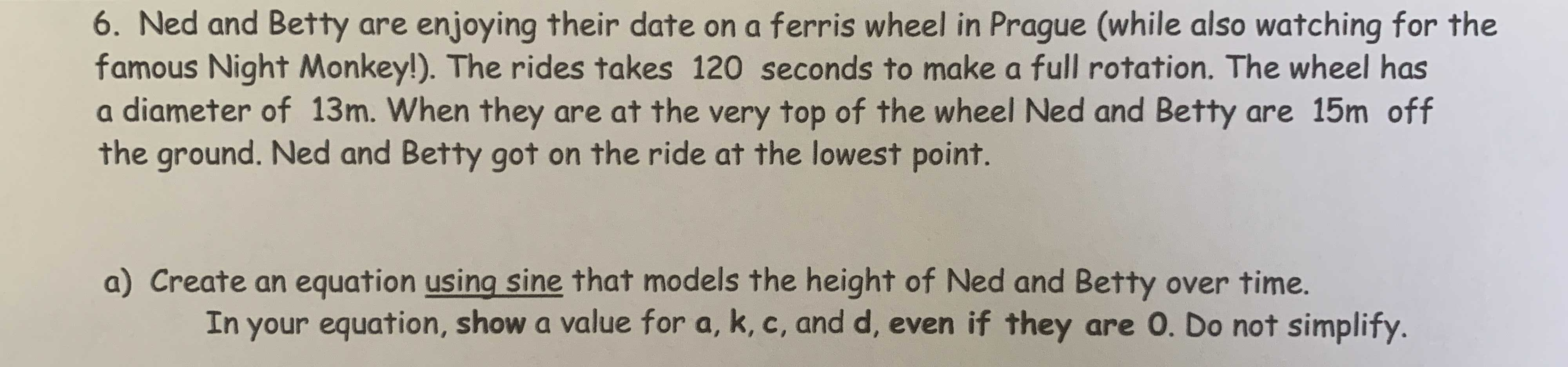### ¿Todavía tienes preguntas de matemáticas?

Pregunte a nuestros tutores expertos
Algebra
Pregunta6. Ned and Betty are enjoying their date on a ferris wheel in Prague (while also watching for the famous Night Monkey!). The rides takes $$120$$ seconds to make a full rotation. The wheel has a diameter of $$13 m$$ . When they are at the very top of the wheel Ned and Betty are $$15 m$$ off the ground. Ned and Betty got on the ride at the lowest point. a) Create an equation using sine that models the height of Ned and Betty over time. In your equation, show a value for $$a , k , c$$ , and $$d$$ , even if they are $$0$$ . Do not simplify.

y=6.5sin($$\frac{\pi (x- 30) }{60}$$)+2